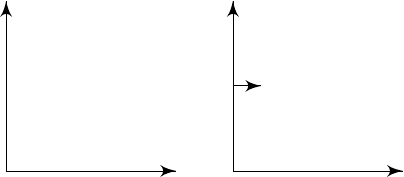1Newtonian dynamics of particles

IA Dynamics and Relativity1.2 Galilean transformations
The goal of this section is to investigate inertial frames. We know that inertial
frames are not unique. Given an inertial frame, what other inertial frames can
we obtain?
First of all, we can rotate our axes or move our origin. In particular, we can
perform the following operations:
Translations of space:
r
0
= r r
0
Translations of time:
t
0
= t t
0
Rotation (and reflection):
r
0
= Rr
with R O(3).
These are not very interesting. They are simply symmetries of space itself.
The last possible transformation is uniform motion. Suppose that
S
is an
inertial frame. Then any other frame
S
0
in uniform motion relative to
S
is also
inertial:
S
S
0
y
x
y
0
x
0
v
Assuming the frames coincide at t = 0, then
x
0
= x vt
y
0
= y
z
0
= z
t
0
= t
Generally, the position vector transforms as
r
0
= r vt,
where
v
is the (constant) velocity of
S
0
relative to
S
. The velocity and acceleration
transform as follows:
˙
r
0
=
˙
r v
¨
r
0
=
¨
r
Definition
(Galilean boost)
.
A Galilean boost is a change in frame of reference
by
r
0
= r vt
t
0
= t
for a fixed, constant v.
All these transformations together generate the Galilean group, which de-
scribes the symmetry of Newtonian equations of motion.
Law
(Galilean relativity)
.
The principle of relativity asserts that the laws of
physics are the same in inertial frames.
This implies that physical processes work the same
at every point of space
at all times
in whichever direction we face
at whatever constant velocity we travel.
In other words, the equations of Newtonian physics must have Galilean
invariance.
Since the laws of physics are the same regardless of your velocity, velocity
must be a relative concept, and there is no such thing as an “absolute velocity”
that all inertial frames agree on.
However, all inertial frames must agree on whether you are accelerating or
not (even though they need not agree on the direction of acceleration since you
can rotate your frame). So acceleration is an absolute concept.